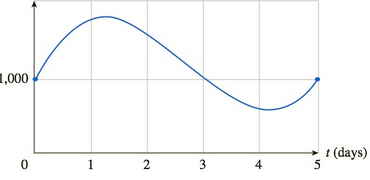Chapter 10.4, Problem 41EFinite Mathematics and Applied Cal...

7th Edition
Stefan Waner + 1 other
ISBN: 9781337274203

Solutions

Chapter
SectionFinite Mathematics and Applied Cal...

7th Edition
Stefan Waner + 1 other
ISBN: 9781337274203
Textbook Problem

Market Index Joe Downs runs a small investment company from his basement. Every week, he publishes a report on the success of his investments, including the progress of the Joe Downs Index. At the end of one particularly memorable week, he reported that the index for that week had the value I ( t ) = 1 , 000 + 1 , 500 t − 800 t 2 + 100 t 3 points, where t represents the number of business days into the week; t ranges from 0 at the beginning of the week to 5 at the end of the week. The graph of I is shown below:I(Joe Down Index)On average, how fast and in which direction was the index changing over the first two business days (the interval [ 0 , 2 ] )?[HINT: See Example 3.]

To determine

To calculate: The rate of change in index over the first two business days and its direction, if the index for a week has a value I(t)=1000+1500t800t2+100t3 and the graph of I is shown below.Explanation

Given Information:

The function is I(t)=1000+1500t800t2+100t3 and the graph of I is shown below:

Formula used:

Average rate of change of f(x) over the interval [a,b] is:

Average rate of change of f=Change in fChange in x=ΔfΔx=f(b)f(a)ba

Calculation:

Consider the function I(t)=1000+1500t800t2+100t3,

From the graph shown below, find the value of index change in first two business day, that is in the interval [0,2].

Apply the average rate of change formula:

Average rate of change of I=I(2)I(0)20

Now, find I(2) and I(0):

Substitute the value 2 in the function I(t)=1000+1500t800t2+100t3:

I(2)=1000+1500(2)800(2)

Still sussing out bartleby?

Check out a sample textbook solution.

See a sample solution

The Solution to Your Study Problems

Bartleby provides explanations to thousands of textbook problems written by our experts, many with advanced degrees!

Get Started

Rationalize Numerator Rationalize the numerator. 94. xx+hhxx+h

Precalculus: Mathematics for Calculus (Standalone Book)

6x2(3x2+2)+14(x+2)

Applied Calculus for the Managerial, Life, and Social Sciences: A Brief Approach

True or False: n=1n+n3n2/3+n3/2+1 is a convergent series.

Study Guide for Stewart's Single Variable Calculus: Early Transcendentals, 8th

For

Study Guide for Stewart's Multivariable Calculus, 8th